# Chapter 10 Self Test

## Catalyst Decay in a Batch Reactor

The elementary irreversible gas phase catalytic reaction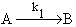is carried out isothermically in a batch reactor. The catalyst deactivation follows a first order decay law and is independent of the concentrations of both A and B.

CA0 = 1 mol/dm3

V0 = 1 dm3

W = 1 kg

kd = 0.1 min-1 at 300 K                 Ed/R = 2,000 K

k1 =0.2 dm3/(kg cat · min) at 300 K Ea/R = 500 K

Hint 1 Write the algorithm and determine a general expression for catalyst activity as a function of time.

Hint 2 Make a qualitative sketch of catalyst activity as a function of time. Does a(t) ever equal zero for a first order decay law?

Hint 3 Write out the general algorithm and derive an expression for conversion as a function of time, the reactor parameters and the catalyst arameters.

Fill in the following algorithm

Mole balance

Rate Law

Decay Law

Stoichiometry

Combine

Solve

1) Separate

2) Integrate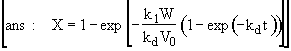Hint 4 Calculate the conversion and catalyst activity in the reactor after 10 minutes at 300 K.

Full Solution

Hint 1. Algorithm and a(t)

Batch–

Mole Balance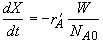Rate Law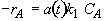Decay Law

First-order decay law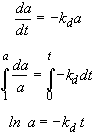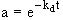Back to Hints

Back to Problem

Hint 2

Sketch a(t)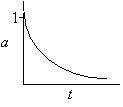a(t) never equals zero for a first-order decay law

Back to Hints

Back to Problem

Hint 3.  Determine X(t)

Stoichiometry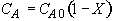Combine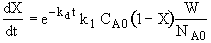Separate and Integrate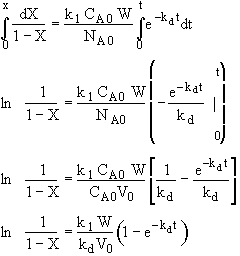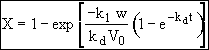where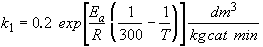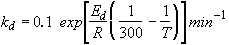Back to Hints

Back to Problem

Hint 4

Calculate conversion after 10 minutes

300 K – No temperature correction needed for k1, kd since T = T1

Results the same for both catalysts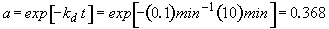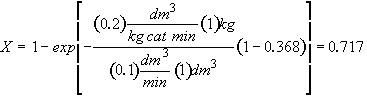For both catalysts 1 and 2 at 300 K

Back to Hints

Back to Problem

# Full Solution

Hint 1. Algorithm and a(t)

Batch–

Mole BalanceRate LawDecay Law

First-order decay lawHint 2

Sketch a(t)a(t) never equals zero for a first-order decay law

Hint 3.  Determine X(t)

StoichiometryCombineSeparate and IntegratewhereHint 4

Calculate conversion after 10 minutes

300 K – No temperature correction needed for k1, kd since T = T1

Results the same for both catalystsFor both catalysts 1 and 2 at 300 K